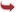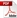International Journal of Scientific and Research Publications

#### IJSRP, Volume 7, Issue 4, April 2017 Edition [ISSN 2250-3153]Radius, Diameter and Center of a Directed Fuzzy Graph Using Algorithm
Dr.K.UMA
Abstract: In this paper, the length of an edge sequence is said to be the number of edges contained in the edge sequence. The length of a fuzzy chain is the number of edges that makes up the fuzzy chain. The distance between vertex i and vertex j is sij, which is defined as the length of the smallest fuzzy chain between vi and vj. The concept of radius and diameter are associated with connected fuzzy graph. The radius of a fuzzy graph is defined as the set of maximum values selected from each row of the distance matrix is in a sense a measure of the closeness of a fuzzy graph. The diameter of a graph is the greatest distance that can be found in the fuzzy graph. To determine the diameter, select the maximum value from each row in the distance matrix. The largest of these numbers is the diameter.
[VIEW FULL PAPER][DOWNLOAD]

Reference this Research Paper (copy & paste below code):

Dr.K.UMA (2017); Radius, Diameter and Center of a Directed Fuzzy Graph Using Algorithm; Int J Sci Res Publ 7(4) (ISSN: 2250-3153). http://www.ijsrp.org/research-paper-0417.php?rp=P646307